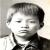## 问个运算符的问题（老问题）

hphper 发布于 2013/11/03 17:20

```int i=5,j=5,p,q;

p=(i++)+(i++)+(i++);
q=(++j)+(++j)+(++j);
printf("%d,%d,%d,%d\n",p,q,i,j);
//结果15,22,8,8```

0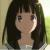0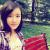0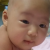0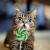win VS 2010 的出来的也是24.

q=(++j)+(++j)+(++j);

j自加三次，可能由于编译问题 q = 8 + 8 + 8。

0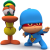0

0